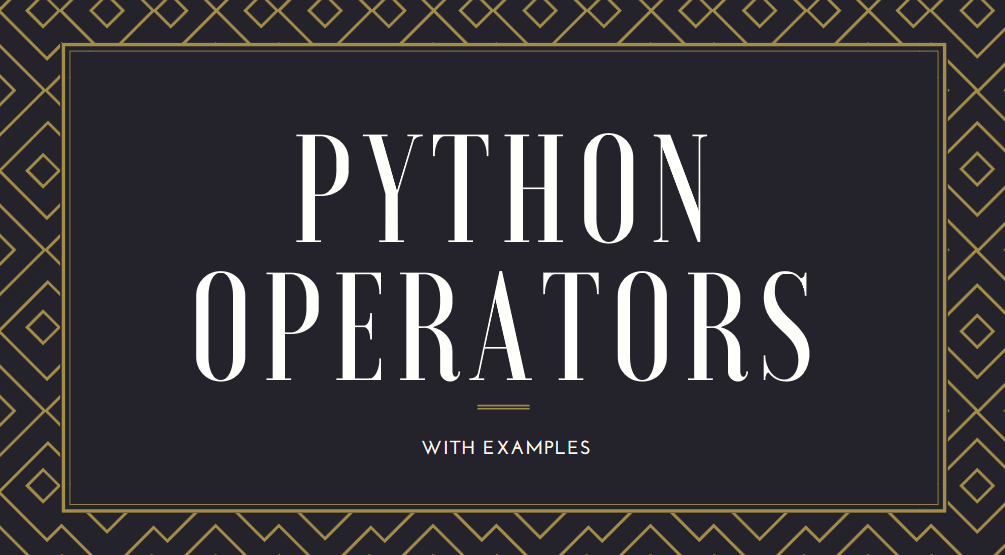# Python Operators and their ExamplesPython is a portable, interpreted, object-oriented programming language. It combines remarkable power with very clear syntax. Moreover, its high-level built-in data structures, combined with dynamic typing and dynamic binding, make it very attractive for rapid application development.

Python is a free, open-source, general-purpose, interpreted, and powerful scripting language for web applications. It is an easy yet powerful programming language that provides structure and support for large applications as well as the power and complexity of traditional high-level languages. Python is the ideal choice if you require a single language with the features of both an interpreted and a scripting language.

Operators play an important part in performing calculations. Besides arithmetic operators, python also supports conditional operators for making value comparisons.

Operators are functionality that do something and can be represented by symbols such as + or by special keywords. Operators require some data to operate on and such data are called operands.

Here are  the complete list of operators which python supports:

S.No
Operator
Name
Explanation Examples
1
+
Plus
Adds the two objects 3+5 gives 8.
‘a’+’b’ gives ‘ab’.
2
Minus
Either gives a negative number or gives the subtraction of one number from the other -5.2 gives a negative number.
50-24 gives 26.
3
*
Multiply
Gives the multiplication of the two numbers or returns the string repeated that many times 2*3 gives 6.
‘la’&’3’ gives ‘lalala’.
4
**
Power
Returns x to the power of y 3**4 gives 81 (i.e. 3*3*3*3)
5
/
Divide
Divide x by y 4/3 gives 1 (Division of integers gives an integer).
4.0/3 or 4/3.0 gives 1.3333333333333333.
6
//
Floor Division
Returns the floor of the quotient 4 // 3.0 gives 1.0
7
%
Modulo
Returns the remainder of the division 8%3 gives 2.

25.5%2.25 gives 1.5
8
<<
Left Shift
Shifts the bits of the number to the left by the number of bits specified. (Each number is represented in memory by bits or binary digits i.e. 0 and 1) 2 << 2 gives 8.
– 2 is represented by 10 in bits.
Left shifting by 2 bits gives 1000 which represents the decimal 8.
9
>>
Right Shift
Shifts the bits of the number to the right by the number of bits specified. 11 >> 1 gives 5
– 11 is represented in bits by 1011 which when right shifted by 1 bit gives 101 which is nothing but decimal 5.
10
&
Bit-wise AND
Bitwise AND of the numbers 5 & 3 gives 1.
11
|
Bit-wise OR
Bitwise OR of the numbers 5 | 3 gives 7
12
^
Bit-wise XOR
5^3 gives 6
13
~
Bit-wise invert
The bitwise inversion of x is -(x+1) ~5 gives -6.
14
<
Less Than
Returns whether x is less than y. All comparison operators return 1 for true and 0 for false. This is equivalent to the special variables True and False respectively.
Note the capitalization of these variables names.
5 < 3 gives 0 (i.e. False) and 3 < 5 gives 1 (i.e. True).
Comparisons can be chained arbitrarily: 3 < 5< 7 gives True.
15
>
Greater Than
Returns whether x is greater than y 5 < 3 returns True.
If both operands are numbers, they are first converted to a common type. Otherwise, it always returns False.
16
<=
Less Than or Equal To
Returns whether x is less than or equal to y x = 3; y = 6; x<= y returns True.
17
>=
Greater Than or Equal To
Returns whether x is greater than or equal to y x = 4; y = 3; x >= 3 returns True.
18
==
Equal To
Compares if the objects are equal x = 2; y = 2; x == y returns True.
x = ‘str’; y = ‘stR’; x == y returns False.
x = ‘str’; y = ‘str’; x == y returns True.
19
!=
Not Equal To
Compares if the objects are not equal x = 2; y = 3; x != y returns True.
20
not
Boolean NOT
If x is True, it returns False. If x is False, it returns True. x = True; not y returns False.
21
and
Boolean AND
x and y returns False if x is False, else it returns evaluation of y x = False; y = True; x and y returns False since x is False.
In this case, Python will not evaluate y since it knows that the value of the expression will has to be false (since x is False). This is called short-circuit evaluation.
22
or
Boolean OR
If x is True, it returns True, else it returns evaluation of y x = True; y = False; x or y returns True.
Short-circuit evaluation applies here as well.